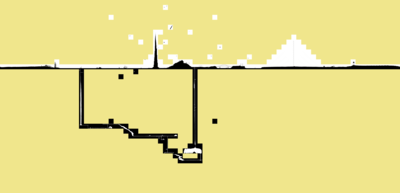# Map for xkcd No:1110

2012-09-21

Three times a week, Randall Munroe draws a new comic entry on xkcd.com. This Wednesday, the comic was quite unusual and pretty awesome: xkcd #1110. Basically, it's a giant map browsable with a limited field of view. I played with it for something like 12 seconds, and then decided to create a map so I wouldn't miss anything. Oh, just like pokanalysis...

This article is pretty accessible from a technical point of view, and I know a lot of other people did that as well, but I was willing to share the method I used anyway.

First, we need to grab all the images to process them locally.

We're going to use several scripts, so we need a place for some common code, let's say in a `xkcdcommon.py` script:

``````class XKCD1110:

def __init__(self):
self.size = [14, 48, 25, 33]
self.w = self.size + self.size
self.h = self.size + self.size

def tile_name(self, x, y):
x -= self.size
y -= self.size
return (('%d%c' % (y + 1, 's')) if y >= 0 else ('%d%c' % (-y, 'n'))) + \
(('%d%c' % (x + 1, 'e')) if x >= 0 else ('%d%c' % (-x, 'w')))
``````

This is a direct mapping of the code in 1110.js.

We now know the map has `width=48+33=81` and `height=14+25=39`. We also have a function to map the `[x;y]` position to the segmented image. Each image has a size of `2048x2048`. This is a pretty huge map we have there.

Since there is no listing of the images available, we will have to brute-force the download (if someone has another trick I'm interested). Here is the `img-url-list.py` script to print the full list of the potential images on stdout:

``````#!/usr/bin/env python

from xkcdcommon import XKCD1110

xkcd = XKCD1110()
for y in range(xkcd.h):
for x in range(xkcd.w):
print "http://imgs.xkcd.com/clickdrag/%s.png" % xkcd.tile_name(x, y)
``````

And we can download them in a `pic/` directory with the following `download-pic.sh` shell script:

``````#!/bin/sh

mkdir -p pic
for url in `./img-url-list.py`; do
wget -c "\$url" -P pic
sleep .5
done
``````

To simplify the reconstruction of the map, we might want to use a padding tile, let's generate one in a special color (to better mark the difference with the other tiles):

``````% convert -size 2048x2048 canvas:khaki pic/ref.png
``````

## Make the small tiles

The map is really large and we want to construct a preview, so we need a `reduce-images.sh` script to reduce each downloaded images in `pic/`:

``````#!/bin/sh

[ \$# -ne 1 ] && printf "usage: \$0 size\n" && exit 0

mkdir -p small
cd pic
for f in *.png; do
convert \$f -resize \${1}x\${1} ../small/\$f
done
``````

This will fill the `small/` directory with the reduced images (`2048x2048` to `64x64` if the run script parameter is `64`).

## Complete map image output

Now for our first output, we can construct a full picture of the reduced images using again ImageMagick. Here is a `output-image.py` python script to generate the appropriate `convert` command line to stack all the images into one:

``````#!/usr/bin/env python

import os.path
from xkcdcommon import XKCD1110

xkcd = XKCD1110()

cmd = 'convert'
for y in range(xkcd.h):
cmd += ' ('
for x in range(xkcd.w):
tile = 'small/' + xkcd.tile_name(x, y) + '.png'
if not os.path.isfile(tile):
tile = 'small/ref.png'
cmd += ' %s' % tile
cmd += ' +append )'
cmd += ' -append xkcd.png'
print(cmd)
``````

Pretty easy to use:

``````% \$(./output-image.py)
``````

And here is the `xkcd.png` output:## Complete map in HTML

Now it would be interesting to be able to zoom on a given part, so here is a `output-html.py` script to get a HTML output with clickable images:

``````#!/usr/bin/env python

import sys, os.path
from xkcdcommon import XKCD1110

xkcd = XKCD1110()

if len(sys.argv) != 2:
print('usage: %s size' % sys.argv)
sys.exit(0)

size = int(sys.argv)

<title>XKCD #1110 map</title>
for y in range(xkcd.h):
for x in range(xkcd.w):
tile = xkcd.tile_name(x, y) + '.png'
if not os.path.isfile('pic/' + tile):
tile = 'ref.png'
print('<a href="pic/%s" style="left:%dpx; top:%dpx;"><img src="small/%s" alt="" /></a>' % (tile, x*size, y*size, tile))
print('</body></html>')
``````

That script needs the sizes of the small pictures. Assuming we resized the images with `./resize-images.sh 64`, the HTML page can be generated with:

``````% ./output-html.py 64 > xkcd1110.html
``````

The output of the page is viewable here: interactive xkcd #1110 full map.

That's all folks.